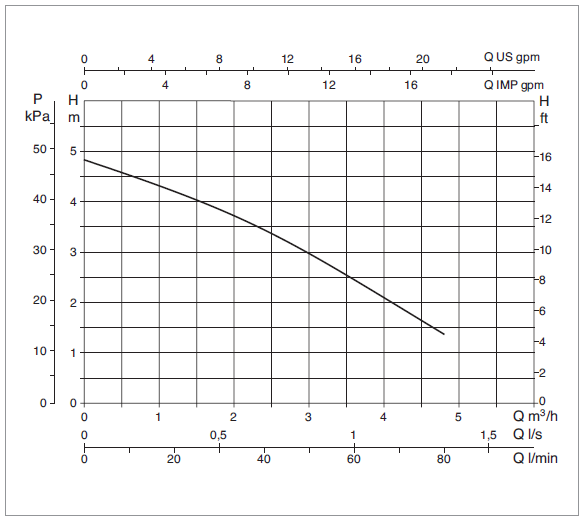# How to select pump

Posted by admin 19/11/2019 0 Comment(s)

To size a water pump, all we need is flowrate and height. Let's find out how to get these information.

Determine the height of water to lift (Pressure head)

Use measuring tape to measure height from pump to water height you want to lift. This measurement obtained is called "pressure head"

Determine the amount the water to transfer (Flowrate).

For circulate water in a fish pond, it is recommended that pond water is circulated at least once per hour. To calculate the flowrate, please see step 1.

Step 1

Calculate volume of water in pond

Cuboid shape pond (m3) = Length (m) x Width (m) x Height (m)

Cylinder shape pond (m3) = 3.142 x Radius (m) x Radius (m) x Height (m)

Step 2

Determine flowrate

Note: It is recommended that pond water is circulated at least once per hour.

Therefore,

Litres of water in pond = Flowrate (L/H)

Convert litres/hour (L/H) to litres/min (L/min) = Flowrate (L/H) / 60

Step 3

Use result obtained at Step 2 to select a pump.

1.  If the water doesn't need to be lifted to height more that 0.5m, just select a pump that have 'max. output' or flowrate within the value obtained in step 2
2. If the water need to lift higher than 0.5 meter, make sure the pump able to lift water to the height you need by select the pump with the right Pressure head. Look at pump performance chart  'Pressure.Head vs Flowrate" like picture below. Ensure the pump can lift water to the height you required while able to move at the flowrate obtained in step 2. Pump performance chart that can be found at product page.Step 4

Find pumps by using our pump finder in here.

Tags: pump sizing